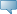## Catalog Entries

Current
Feb 22, 2020Select the Course Number to get further detail on the course. Select the desired Schedule Type to find available classes for the course.

 MAT 310 - Applied Linear Algebra Applied Linear Algebra Prerequisites: MAT 230, MAT 243, MAT 244 This course covers systems of linear equations and matrix algebra with emphasis on applications. Topics include systems of linear equations and their solutions, matrices and matrix algebra, determinants and inverse matrices, vector spaces and subspaces, linear independence, bases for vector spaces, dimension, linear transformations, inner products, orthogonal bases and projections, eigenvalues and eigenvectors. Possible areas of applications include graph theory, coding and curve fitting. Use of technology is integral to the course. 3.000 Credit hours 3.000 Lecture hours Levels: Undergraduate Schedule Types: Lecture Mathematical Sciences Department